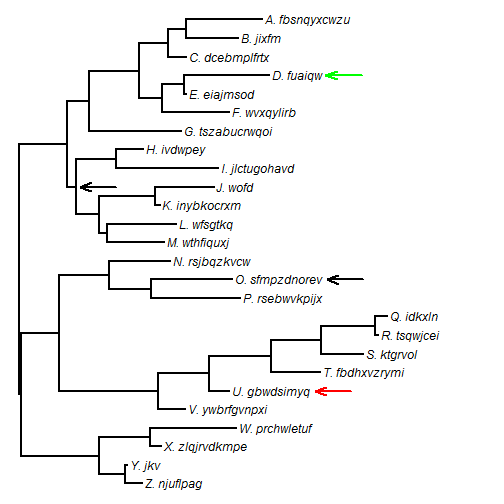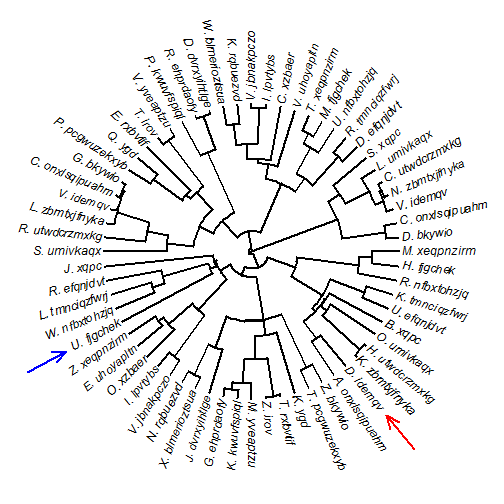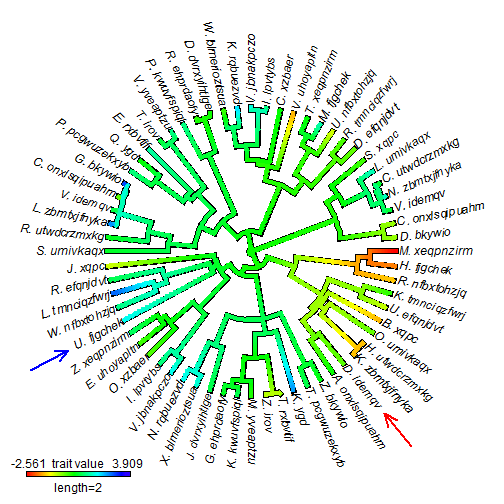Friday, December 26, 2014

Wrapper function to optimize the λ tree transformation for a discrete trait

Today I responded to an R-sig-phylo query by posting some code to optimize Pagel's λ tree transformation for a discrete character evolving by a continuous-time Markov chain. I did this by writing a very simple wrapper around ape's ace function for ancestral character estimation, which also fits this model:

## here's the wrapper function
fitLambda<-function(tree,x,model="ER"){
lik<-function(lambda,tree,x,model)
logLik(ace(x,rescale(tree,model="lambda",lambda),
type="discrete",model=model))
obj<-optimize(lik,c(0,1),tree=tree,x=x,model=model,maximum=TRUE)
fit<-ace(x,rescale(tree,model="lambda",lambda=obj\$maximum),
type="discrete",model=model)
I<-fit\$index.matrix
fitted.Q=matrix(fit\$rates[I],dim(I),dim(I),
dimnames=list(dimnames(fit\$lik.anc)[],
dimnames(fit\$lik.anc)[]))
diag(fitted.Q)<--rowSums(fitted.Q,na.rm=TRUE)
list(Q=fitted.Q,lambda=obj\$maximum,logLik=logLik(fit))
}
library(geiger)
library(phytools)
## simulate some data to test it
tree<-pbtree(n=200,scale=1)
Q<-matrix(c(-1,1,1,-1),2,2)
rownames(Q)<-colnames(Q)<-letters[1:2]
x<-sim.history(rescale(tree,model="lambda",lambda=0.7),Q)\$states
## Done simulation(s).
fitLambda(tree,x)
## \$Q
##         a       b
## a -0.7995  0.7995
## b  0.7995 -0.7995
##
## \$lambda
##  0.5154
##
## \$logLik
##  -128.6

Note that the same model can also be fit using geiger's fitDiscrete function, but the poster reported some issues with convergence which made me want to post the second way. Here is fitDiscrete

fitDiscrete(tree,x,transform="lambda")
## DLSODA-  Warning..Internal T (=R1) and H (=R2) are
##       such that in the machine, T + H = T on the next step
##      (H = step size). Solver will continue anyway.
## In above message, R1 = 0, R2 = 0
##
## DINTDY-  T (=R1) illegal
## In above message, R1 = 2.8036e-222
##
##       T not in interval TCUR - HU (= R1) to TCUR (=R2)
## In above message, R1 = 0, R2 = 0
##
## DINTDY-  T (=R1) illegal
## In above message, R1 = 8.66551e-221
##
##       T not in interval TCUR - HU (= R1) to TCUR (=R2)
## In above message, R1 = 0, R2 = 0
##
## DLSODA-  Trouble in DINTDY.  ITASK = I1, TOUT = R1
## In above message, I1 = 1
##
## In above message, R1 = 8.66551e-221
##

Here I've excluding a whole bunch of error messages....

##
## In above message, R1 = 8.66551e-221
##
## GEIGER-fitted comparative model of discrete data
##  fitted Q matrix:
##             a       b
##     a -0.7928  0.7928
##     b  0.7928 -0.7928
##
##  fitted 'lambda' model parameter:
##  lambda = 0.508644
##
##  model summary:
##  log-likelihood = -128.569446
##  AIC = 261.138891
##  AICc = 261.199805
##  free parameters = 2
##
## Convergence diagnostics:
##  optimization iterations = 100
##  failed iterations = 56
##  frequency of best fit = NA
##
##  object summary:
##  'lik' -- likelihood function
##  'bnd' -- bounds for likelihood search
##  'res' -- optimization iteration summary
##  'opt' -- maximum likelihood parameter estimates

Also, fitting the λ model to discrete trait data has always struck me as a somewhat peculiar enterprise, so this is posted without any prejudice towards whether this is a good idea, a bad idea, or an average idea in the first place!

Monday, December 15, 2014

New (non-CRAN) version of phytools; more on new phytools function add.arrow

I just posted a new non-CRAN version of phytools (go here and pick the latest version, which can be installed from source). I'm working towards an update to phytools on CRAN; but I need to do some more debugging of phytools' new functions, such as locate.yeti and locate.fossil, as well as add some details to new documentation, before I do that.

This latest version now includes the aforementioned locate.fossil. It also includes fitPagel, a relatively simple wrapper function that implements Pagel's 1994 method to test for correlated evolution of two binary characters, as well as the brand new function add.arrow, which adds an arrow to a plotted radial or square phylogram.

Here's a quick demo of the last function in this list:

library(phytools)
packageVersion("phytools")
##  '0.4.42'
## simulate tree with realistic tip labels
set.seed(10)
tree<-rtree(n=26)
tree\$tip.label<-paste(LETTERS[26:1],"._",sep="",
replicate(26,paste(sample(letters,sample(3:12,1)),
collapse="")))
h<-max(nodeHeights(tree))
plotTree(tree,ftype="i")
## we can do it without supplying a tree as a function argument
## we can have an arrow point to a nodeOf course, as described in the original post the function also works for radial (type="fan") trees, e.g.:

tree<-pbtree(n=64)
tree\$tip.label<-paste(sample(LETTERS,64,replace=TRUE),"._",sep="",
replicate(26,paste(sample(letters,sample(3:12,1)),
collapse="")))
h<-max(nodeHeights(tree))
plotTree(tree,type="fan",ftype="i")
## setEnv=TRUE for this type is experimental. please be patient with bugsAnd we can use it for plotted objects of class "contMap" and "densityMap", e.g.:

x<-fastBM(tree)
obj<-contMap(tree,x,plot=FALSE)
plot(obj,type="fan",legend=2)